# Python编程基础

• Python是一个结合了解释性、编译性、互动性和面向对象的高级程序设计语言，结构简单，语法定义清晰。
• Python最具特色的就是使用缩进来表示代码块，不需要使用大括号{}。
• 缩进的空格数是可变的，但是同一个代码块的语句必须包含相同的缩进空格数。
```answer = int(input("请输入一个整数："))
print("hello,")
print("it's True")
else:
print("sorry,")
print("it's False")
```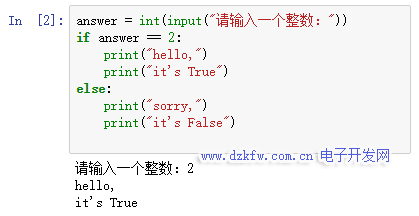1、基础数据类型
• Python3中有六个标准的数据类型：Number（数字）、String（字符串）、List（列表）、Tuple（元组）、Set（集合）、Dictionary（字典）。其中，不可变数据类型有：Number、String、Tuple；可变数据类型有：List、Dictionary、Set。
• Python3支持的数字类型有int（整数）、float（浮点数）、bool（布尔型）、complex（复数）四种类型。
2、变量和赋值
• Python 中的变量是不需要声明数据类型的，变量的“类型”是所指的内存中被赋值对象的类型。
• 同一变量可以反复赋值，而且可以是不同类型的变量，这也是Python语言称之为动态语言的原因。
• Python允许同时为多个变量赋值。
```brower = 'Google'  #字符串类型
brower = 100  #整数类型
brower = 123.45  #浮点数类型
brower = 2 + 3j  #复数类型
```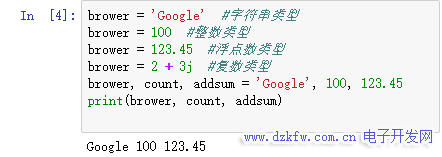3、操作符和表达式
4、字符串
• 字符串被定义为引号之间的字符集合，在Python中，字符串用单引号(’), 双引号("), 三引号(’’’)括起来，且必须配对使用。
• 当Python字符串中有一个反斜杠时表示一个转义序列的开始，称反斜杠为转义符。
44
字符串的运算

5、流程控制

1.分支结构：

Python语言中使用关键字if、elif、else来表示，基本语法格式如下：

```if condition：
if-block
[elif condition:
elif-block
else:
else-block]
```

2. 循环语句

（1）while循环

```while语句语法格式：
while condition:
while-block
```

```i = 0
while i < 20:
if i % 3 == 0:
print(i,end= " ")
i = i + 1
```
``` for  v  in  Seq：
for_block
```

```sum = 0
for i in range(1,6):
sum = sum + i
print("1+2+3+4+5 = %d"%sum)
```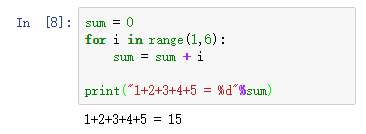1、列表
• 列表是Python中最具灵活性的有序集合对象类型。和字符串不同的是，列表具有可变长度、异构以及任意嵌套列表的特点。
• 列表是可变对象，支持在原处修改。

1. L.append(v) ：把元素v添加到列表L的结尾，相当于a[len(a)] = [v]
2. L.insert(i,v)：将值v插入到列表L的索引i处
3. L.index(x)：返回列表中第一个值为x的元素的索引
4. L.remove(v)：从列表L中移除第一次找到的值v
5. L.pop([i])：从列表的指定位置删除元素，并将其返回。如果没有指定索引，a.pop()返回最后一个元素。
6. L.reverse()：倒排列表中的元素
7. L.count(x)：返回x在列表中出现的次数
8. L.sort(key=None, reverse=False)：对链表中的元素进行适当的排序。```vec = [2, 4, 6, 8, 10]
print([3*x for x in vec])
vec = [2, 4, 6,8,10]
print([3*x  for  x  in  vec  if x > 6])
vec1 = [2, 4, 6]
vec2 = [4, 3, -9]
print([x*y for x in vec1 for y in vec2 if x*y>0])
```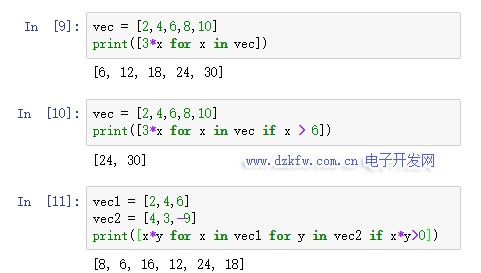2、元组
• 元组有很多用途，例如：坐标(x, y)，数据库中的员工记录等等。
• 元组和字符串一样，不可改变，即不能给元组的一个独立的元素赋值。
• 元组和列表看起来不同的一点是：元组用的是圆括号（），而列表用的是方括号[]。
```tup = tuple('bar')  #创建元组
print('输出元组tup:',tup)  #输出元组
nested_tup = (4,5,6),(7,8)
print('输出元组图tup:',nested_tup)#输出元素是元组的元组
print('元组的连接',tup+tuple('wwy'))
a,b,c = tup #元组的拆分
print(a,b,c)
print(tup.count(a)) # 统计某个数值在元组中出现的次数
```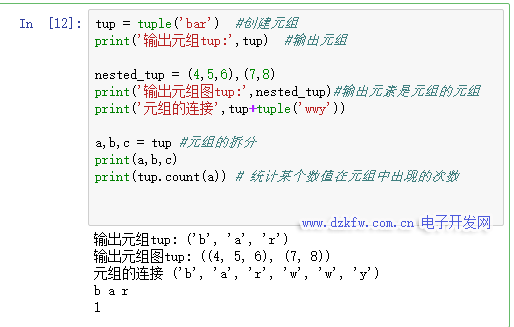3、字典
• 字典，也称映射，是一个由键/值对组成的非排序可变集合体。键值对在字典中以下面的方式标记：
• dict = {key1 : value1,key2 : value2 }
• 键/值对用冒号分割，而各个元素之间用逗号分割，所有元素都包括在花括号中。值得注意的是，字典中的键必须是唯一的，只能使用不可变的对象（比如字符串）来作为字典的键，字典中的键/值对是没有顺序的。
```scientists = {'Newton' : 1642, 'Darwin' : 1809, 'Turing' : 1912}
print('keys:', scientists.keys())  #返回字典中的所有键
print('values:', scientists.values()) #返回字典中的所有值
print('items:', scientists.items()) #返回所有键值对，形式(键, 值)
print('get:', scientists.get('Curie', 1867))  # get方法
temp = {'Curie' : 1867, 'Hopper' : 1906, 'Franklin' : 1920}
scientists.update(temp)  #用字典temp更新字典scientists
print('after update:', scientists)
scientists.clear() # 清空字典
print('after clear:', scientists)
```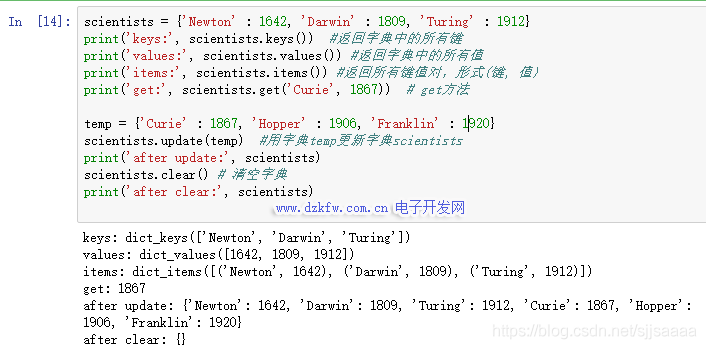4、集合
• 集合是一个由唯一元素组成的非排序集合体。
• 集合中的元素没有特定顺序，集合中没有重复项。
• 可以使用大括号{ }或者set()函数创建集合，但是，创建一个空集合必须用 set()，因为{ }是用来创建一个空字典。
```set1 = set([0,1,2,3,4])
set2 = set([1,3,5,7,9])
print(set1.issubset(set2))
print(set1.union(set2))
print(set2.difference(set1))
print(set1.issubset(set2))
```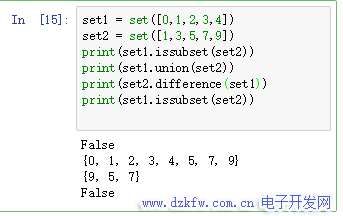• 函数是对程序逻辑进行过程化和结构化的一种方法，函数最大的优点是增强了代码的重用性和可读性。
• Python不但能灵活地定义函数，而且本身内置了很多有用的函数，可以直接调用。

```def  function_name(arguments):
function_block
```

1. 函数代码块以 def 关键词开头，后接函数标识符名称和圆括号()；
2. function_name是用户自定义的函数名称；
3. arguments是零个或多个参数，且任何传入参数必须放在圆括号内；
4. 最后必须跟一个冒号 :，函数体从冒号开始，并且缩进；
5. function_block实现函数功能的语句块。

lambda函数
Python使用lambda来创建匿名函数,准确地说，lambda只是一个表达式，函数体比def定义的函数简单的多，在lambda表达式中只能封装有限的逻辑。除此之外，lambda函数拥有自己的命名空间，且不能访问自有参数列表之外或全局命名空间里的参数。

```polynominal = lambda x,y,z: 1+2*x+y**2+z*y
polynominal(1,2,3)
```1. 打开文件：open()函数；
3. 对读取到的数据进行处理；
4. 关闭文件：close()。

file_object = open(file_name [, access_mode = “r”, buffering= -1])

2、数据的读取方法
```f = open("记事本.txt",mode = 'r')
print(content)
f.close()
print(type(content))

f = open("记事本.txt",mode='r')
print(content)
f.close()
```
3、读取csv文件

CSV (Comma Separated Values)，即逗号分隔值，也称为字符分隔值，因为分隔符除了逗号，还可以是制表符，是一种常用的文本格式，用以存储表格数据，包括数字或者字符。

CSV文件具有如下特点：

1. 纯文本，使用某个字符集，比如ASCII、Unicode或GB2312；
2. 以行为单位读取数据，每行一条记录；
3. 每条记录被分隔符分隔为字段；
4. 每条记录都有同样的字段序列。

Python内置了csv模块，import csv之后就可以读取CSV文件了。

```import csv
with open("student.csv", "r") as f:
rows = [row for row in reader]
for item in rows:
print(item)
```
4、文件写入与关闭

• write() 函数用于向文件中写入指定字符串，同时需要将open函数中文件打开的参数设置为 mode = w。
• write() 是逐次写入，writelines() 可将一个列表中的所有数据一次性写入文件。
• 如果有换行需要，则要在每条数据后增加换行符，同时用“字符串 .join() ”的方法将每个变量数据联合成一个字符串，并增加间隔符 “\t”。

```import csv
content = [['0','hanmei','23','81'],
['1','mayi','18','99']]
f = open("test.csv","w",newline='')#如果不加newline=""，就会出现空行
content_out = csv.writer(f)#生成writer对象存储器
for con in content:
content_out.writerow(con)
f.close()
```

##### Tags：Python,编程基础

• 上一篇文章：
• 下一篇文章： 没有了
• 请文明参与讨论，禁止漫骂攻击。 昵称：注册  登录
网友评论
热门文章
• 此栏目下没有热点文章